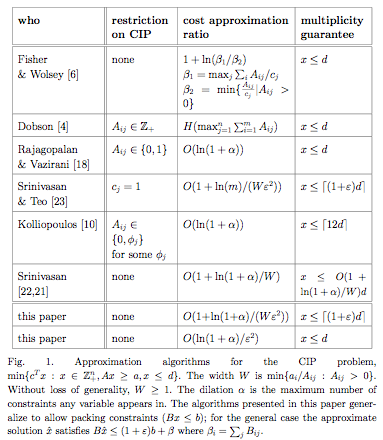## neal young / Kolliopoulos05Approximation

•Given matrices A and B and vectors a, b, c and d, all with non-negative entries, we consider the problem of minimizing c$$\cdot$$x subject to x$$\in Z^n_+$$, Ax ≥ a, Bx ≤ b, and x ≤ d. We give a bicriteria-approximation algorithm that, given \epsilon$$\in$$(0, 1], finds a solution of cost O(log(m)/\epsilon2) times optimal, meeting the covering constraints Ax≥a and multiplicity constraints (x≤d), and satisfying Bx≤(1 + \epsilon)b + β, where β is the vector of row sums, β_i=$$\sum_j$$ Bij. Here m is the number of rows of A.

This gives an O(log m)-approximation algorithm for CIP --- minimum-cost covering integer programs with multiplicity constraints, i.e., the special case when there are no packing constraints Bx ≤ b. The previous best approximation ratio has been O(log maxj $$\sum_i$$Aij since 1982. CIP contains the set cover problem as a special case, so O(log m)-approximation is the best possible unless P=NP.